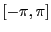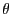Next: Example 3 Up: Examples Previous: Examples   Contents

Example 2

The problem we want to solve has been presented in section 2.3.5.2 and section 15.1.1. The name of the test program is Test_Solve_JH_General1.

If epsilonf=0.01 the program using the maximum equation ordering find the two solutions using 50 boxes.

If we use the single bisection smear mode the program using the maximum equation ordering find the two solutions in 4260ms using 32 boxes.

Note that we may improve the efficiency of the procedure by using simplification procedures such as the 2B (section 2.17) and the 3B method. In that case for epsilonf=1e-6, epsilon=1e-6 the number of boxes will have been reduced to 2 and both solutions will be guaranteed. even with a search space offor.

Jean-Pierre Merlet 2012-12-20